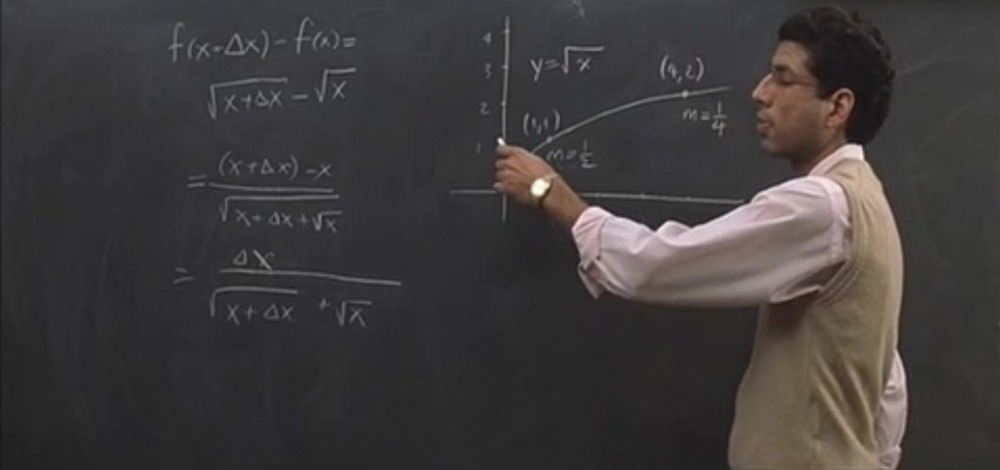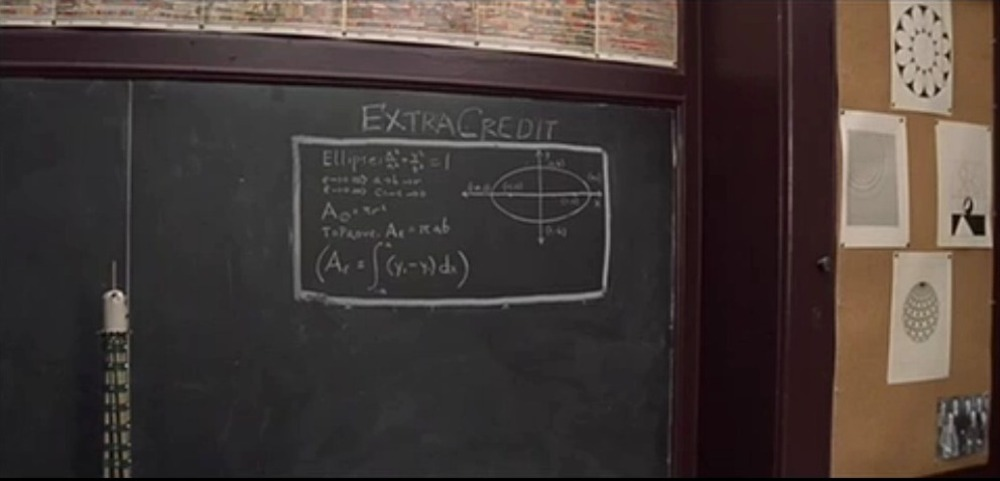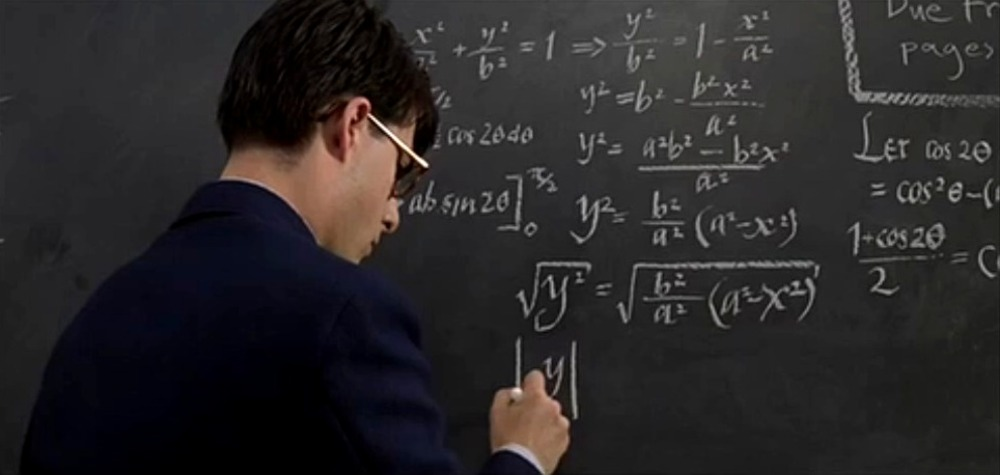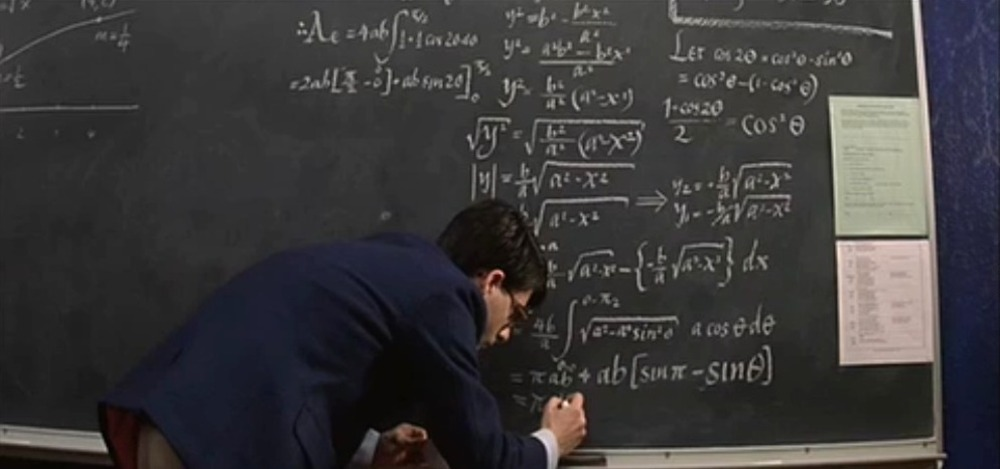# The blackboards of Rushmore

 In the movie Rushmore some blackboards with math appear. Lets look at them: 1) The first blackboard deals with the derivation of the formula d/dx f(x) for the square root function f(x)= x1/2. We have seen that. Multiply both sides with f(x+h)+f(x) to get f(x+h)-f(x) = (f(x+h)2 - f(x)2)/(f(x+h)+f(x) = h/(f(x+h)+f(x). Therefore [f(x+h)-f(x)]/h is equal to 1/[f(x+h) + f(x)] which goes to 1/(2 f(x)) in the limit h to zero. 2) The side blackboard shows an Extra credit problem. Displayed is the ellipse x2/a2 + y2/b2 = 1. The problem is to show that A = int_-aa (y+ - y-) dx= pi a b. [That this is the hardest problem in geometry is of course a joke. The scene is a day dream in a comedy. It can be done easiest with a change of variable reducing it to the circle. In multivariable calculus it is done in polar coordinates or using Greens theorem. In single variable calculus it is a prototype of a problem for trig substitution and this is how it is done on the next blackboards. ] 3) Max Fischer first writes y = (b/a) (a2 - x2)1/2 and then integrates is from -a to a. He substitutes x=a sin(u) to get the integral of b a cos2(t) from 0 to pi/2. He then uses the double angle formula cos2(t) = [1+cos(2t)]/2 to get the area 4 a b int0pi/2 (1/2+cos(2t)/2) dt = pi a b.